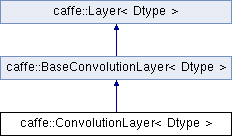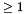Caffe
caffe::ConvolutionLayer< Dtype > Class Template Reference

Convolves the input image with a bank of learned filters, and (optionally) adds biases. More...

`#include <conv_layer.hpp>`

Inheritance diagram for caffe::ConvolutionLayer< Dtype >:## Public Member Functions

ConvolutionLayer (const LayerParameter &param)

virtual const char * type () const
Returns the layer type.Public Member Functions inherited from caffe::BaseConvolutionLayer< Dtype >
BaseConvolutionLayer (const LayerParameter &param)

virtual void LayerSetUp (const vector< Blob< Dtype > *> &bottom, const vector< Blob< Dtype > *> &top)
Does layer-specific setup: your layer should implement this function as well as Reshape. More...

virtual void Reshape (const vector< Blob< Dtype > *> &bottom, const vector< Blob< Dtype > *> &top)
Adjust the shapes of top blobs and internal buffers to accommodate the shapes of the bottom blobs. More...

virtual int MinBottomBlobs () const
Returns the minimum number of bottom blobs required by the layer, or -1 if no minimum number is required. More...

virtual int MinTopBlobs () const
Returns the minimum number of top blobs required by the layer, or -1 if no minimum number is required. More...

virtual bool EqualNumBottomTopBlobs () const
Returns true if the layer requires an equal number of bottom and top blobs. More...Public Member Functions inherited from caffe::Layer< Dtype >
Layer (const LayerParameter &param)

void SetUp (const vector< Blob< Dtype > *> &bottom, const vector< Blob< Dtype > *> &top)
Implements common layer setup functionality. More...

Dtype Forward (const vector< Blob< Dtype > *> &bottom, const vector< Blob< Dtype > *> &top)
Given the bottom blobs, compute the top blobs and the loss. More...

void Backward (const vector< Blob< Dtype > *> &top, const vector< bool > &propagate_down, const vector< Blob< Dtype > *> &bottom)
Given the top blob error gradients, compute the bottom blob error gradients. More...

vector< shared_ptr< Blob< Dtype > > > & blobs ()
Returns the vector of learnable parameter blobs.

const LayerParameter & layer_param () const
Returns the layer parameter.

virtual void ToProto (LayerParameter *param, bool write_diff=false)
Writes the layer parameter to a protocol buffer.

Dtype loss (const int top_index) const
Returns the scalar loss associated with a top blob at a given index.

void set_loss (const int top_index, const Dtype value)
Sets the loss associated with a top blob at a given index.

virtual int ExactNumBottomBlobs () const
Returns the exact number of bottom blobs required by the layer, or -1 if no exact number is required. More...

virtual int MaxBottomBlobs () const
Returns the maximum number of bottom blobs required by the layer, or -1 if no maximum number is required. More...

virtual int ExactNumTopBlobs () const
Returns the exact number of top blobs required by the layer, or -1 if no exact number is required. More...

virtual int MaxTopBlobs () const
Returns the maximum number of top blobs required by the layer, or -1 if no maximum number is required. More...

virtual bool AutoTopBlobs () const
Return whether "anonymous" top blobs are created automatically by the layer. More...

virtual bool AllowForceBackward (const int bottom_index) const
Return whether to allow force_backward for a given bottom blob index. More...

bool param_propagate_down (const int param_id)
Specifies whether the layer should compute gradients w.r.t. a parameter at a particular index given by param_id. More...

void set_param_propagate_down (const int param_id, const bool value)
Sets whether the layer should compute gradients w.r.t. a parameter at a particular index given by param_id.

## Protected Member Functions

virtual void Forward_cpu (const vector< Blob< Dtype > *> &bottom, const vector< Blob< Dtype > *> &top)
Using the CPU device, compute the layer output.

virtual void Forward_gpu (const vector< Blob< Dtype > *> &bottom, const vector< Blob< Dtype > *> &top)
Using the GPU device, compute the layer output. Fall back to Forward_cpu() if unavailable.

virtual void Backward_cpu (const vector< Blob< Dtype > *> &top, const vector< bool > &propagate_down, const vector< Blob< Dtype > *> &bottom)
Using the CPU device, compute the gradients for any parameters and for the bottom blobs if propagate_down is true.

virtual void Backward_gpu (const vector< Blob< Dtype > *> &top, const vector< bool > &propagate_down, const vector< Blob< Dtype > *> &bottom)
Using the GPU device, compute the gradients for any parameters and for the bottom blobs if propagate_down is true. Fall back to Backward_cpu() if unavailable.

virtual bool reverse_dimensions ()

virtual void compute_output_shape ()Protected Member Functions inherited from caffe::BaseConvolutionLayer< Dtype >
void forward_cpu_gemm (const Dtype *input, const Dtype *weights, Dtype *output, bool skip_im2col=false)

void forward_cpu_bias (Dtype *output, const Dtype *bias)

void backward_cpu_gemm (const Dtype *input, const Dtype *weights, Dtype *output)

void weight_cpu_gemm (const Dtype *input, const Dtype *output, Dtype *weights)

void backward_cpu_bias (Dtype *bias, const Dtype *input)

void forward_gpu_gemm (const Dtype *col_input, const Dtype *weights, Dtype *output, bool skip_im2col=false)

void forward_gpu_bias (Dtype *output, const Dtype *bias)

void backward_gpu_gemm (const Dtype *input, const Dtype *weights, Dtype *col_output)

void weight_gpu_gemm (const Dtype *col_input, const Dtype *output, Dtype *weights)

void backward_gpu_bias (Dtype *bias, const Dtype *input)

int input_shape (int i)
The spatial dimensions of the input.Protected Member Functions inherited from caffe::Layer< Dtype >
virtual void CheckBlobCounts (const vector< Blob< Dtype > *> &bottom, const vector< Blob< Dtype > *> &top)

void SetLossWeights (const vector< Blob< Dtype > *> &top)Protected Attributes inherited from caffe::BaseConvolutionLayer< Dtype >
Blob< int > kernel_shape_
The spatial dimensions of a filter kernel.

Blob< int > stride_
The spatial dimensions of the stride.

The spatial dimensions of the padding.

Blob< int > dilation_
The spatial dimensions of the dilation.

Blob< int > conv_input_shape_
The spatial dimensions of the convolution input.

vector< int > col_buffer_shape_
The spatial dimensions of the col_buffer.

vector< int > output_shape_
The spatial dimensions of the output.

const vector< int > * bottom_shape_

int num_spatial_axes_

int bottom_dim_

int top_dim_

int channel_axis_

int num_

int channels_

int group_

int out_spatial_dim_

int weight_offset_

int num_output_

bool bias_term_

bool is_1x1_

bool force_nd_im2col_Protected Attributes inherited from caffe::Layer< Dtype >
LayerParameter layer_param_

Phase phase_

vector< shared_ptr< Blob< Dtype > > > blobs_

vector< bool > param_propagate_down_

vector< Dtype > loss_

## Detailed Description

### template<typename Dtype> class caffe::ConvolutionLayer< Dtype >

Convolves the input image with a bank of learned filters, and (optionally) adds biases.

Caffe convolves by reduction to matrix multiplication. This achieves high-throughput and generality of input and filter dimensions but comes at the cost of memory for matrices. This makes use of efficiency in BLAS.

The input is "im2col" transformed to a channel K' x H x W data matrix for multiplication with the N x K' x H x W filter matrix to yield a N' x H x W output matrix that is then "col2im" restored. K' is the input channel * kernel height * kernel width dimension of the unrolled inputs so that the im2col matrix has a column for each input region to be filtered. col2im restores the output spatial structure by rolling up the output channel N' columns of the output matrix.

## ◆ ConvolutionLayer()

template<typename Dtype >
 caffe::ConvolutionLayer< Dtype >::ConvolutionLayer ( const LayerParameter & param )
inlineexplicit
Parameters
 param provides ConvolutionParameter convolution_param, with ConvolutionLayer options: num_output. The number of filters. kernel_size / kernel_h / kernel_w. The filter dimensions, given by kernel_size for square filters or kernel_h and kernel_w for rectangular filters. stride / stride_h / stride_w (optional, default 1). The filter stride, given by stride_size for equal dimensions or stride_h and stride_w for different strides. By default the convolution is dense with stride 1. pad / pad_h / pad_w (optional, default 0). The zero-padding for convolution, given by pad for equal dimensions or pad_h and pad_w for different padding. Input padding is computed implicitly instead of actually padding. dilation (optional, default 1). The filter dilation, given by dilation_size for equal dimensions for different dilation. By default the convolution has dilation 1. group (optional, default 1). The number of filter groups. Group convolution is a method for reducing parameterization by selectively connecting input and output channels. The input and output channel dimensions must be divisible by the number of groups. For group, the convolutional filters' input and output channels are separated s.t. each group takes 1 / group of the input channels and makes 1 / group of the output channels. Concretely 4 input channels, 8 output channels, and 2 groups separate input channels 1-2 and output channels 1-4 into the first group and input channels 3-4 and output channels 5-8 into the second group. bias_term (optional, default true). Whether to have a bias. engine: convolution has CAFFE (matrix multiplication) and CUDNN (library kernels + stream parallelism) engines.

The documentation for this class was generated from the following files: